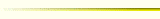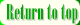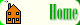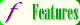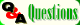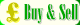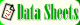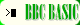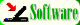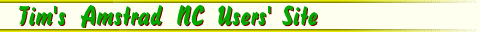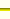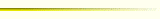By Tim Surtell

Keystroke Dynamics is a method of making computer passwords harder to crack by recording not only the user's password but also the way that the user types it.

An article in 'Electronics -- the Maplin Magazine' entitled "Access control" describes several ways of stopping unauthorized entry into computer systems including signature, hand and eye recognition, but keystroke dynamics is the only method that does not require special equipment.

Because the only thing needed to implement Keystroke Dynamics is a new design of software I decided to experiment and write the necessary program in BBC BASIC for the NC100/200.

Two programs are needed -- one to record the user's entry of the password, and one that can be put in the program that needs to be passworded. The listings of both are shown below. (To see them as text files click on the titles.)

10 DIM kd(3,20),pass(20)
20 CLS
30 thres=70
40 PRINTCHR\$19"Keystroke dynamics password file generator"CHR\$20
60 FORP=1 TO 3
90 NEXT
100 PRINT''"Calculating average keystroke intervals... "
110 FORI=1 TO kdpos-2 STEP 2
120   pass(I)=(kd(1,I)+kd(2,I)+kd(3,I)) DIV 3
130   pass(I+1)=kd(1,I+1)
140 NEXT
150 PRINT:FORI=1 TO kdpos-2 STEP 2:PRINT;pass(I);",";pass(I+1);" ";:NEXT
180 FORI=1 TO kdpos-1
190   PRINT#K,pass(I)
200 NEXT
210 CLOSE#0
220 PRINT'"* Test password. Threshold is ";thres;"%..."'
250 match=0
260 FORI=1 TO kdpos-2 STEP 2
270   IF kd(0,I)=pass(I) OR kd(0,I)-1=pass(I) OR kd(0,I)+1=pass(I) match=match+1
280   IF kd(0,I+1)<>pass(I+1) match=-1:I=kdpos-2
290 NEXT
300 IF match=-1 percent=0 ELSE percent=INT((match/((kdpos-1)/2))*100)
310 PRINTTAB(40)percent;"% -- Access ";:IF percent>320 GOTO230
330 PRINTpercent
340 END
360 FORI=1 TO 20:kd(N,I)=0:NEXT
370 kdpos=1
380 G=INKEY(1)
390 IF G=-1 GOTO380
400 IF G=13 ENDPROC
410 PRINTCHR\$G;
420 IF kdpos=1 kd(N,kdpos)=0:kd(N,kdpos+1)=G:kdpos=kdpos+2:TIME=0:GOTO380
430 kd(N,kdpos)=TIME:kd(N,kdpos+1)=G:kdpos=kdpos+2:TIME=0:GOTO380

PROCkeystroke_dynamics ... 1kb

10 REM ************************
20 REM Keystroke dynamics
30 REM PROCkeystroke_dynamics
40 REM Developed by Tim Surtell
50 REM ************************
60 REM NC100/200 Version 2 70 REM Downloaded from Tim's NC Users' Site
80 REM http://www.gre.ac.uk/~st702/index.htm
90 DIM kd(20):thres=70
120 FORI=1 TO 20:kd(I)=0:NEXT
130 kdpos=1
140 G=INKEY(1)
150 IF G=-1 GOTO140
160 IF G=13 GOTO200
170 PRINTCHR\$G;
180 IF kdpos=1 kd(kdpos)=0:kd(kdpos+1)=G:kdpos=kdpos+2:TIME=0:GOTO140
190 kd(kdpos)=TIME:kd(kdpos+1)=G:kdpos=kdpos+2:TIME=0:GOTO140
210 match=0
220 FORI=1 TO kdpos-2 STEP 2
230   INPUT#K,time,key
240   IF kd(0,I)=time OR kd(0,I)-1=time OR kd(0,I)+1=time match=match+1
250   IF kd(0,I+1)<>key match=-1:I=kdpos-2
260 NEXT
270 IF match=-1 percent=0 ELSE percent=INT((match/((kdpos-1)/2))*100)
280 PRINT"Access ";:IF percent thres PRINT;"denied.":GOTO110 ELSE PRINT;"granted."
290 CLOSE#K
300 ENDPROC

Program analysis

I will now describe the operation of the password recorder program. The other program (PROCkeystroke_dynamics) is similar to PROCenter_password in the password recorder program.

As each key is pressed two things need to be recorded:

• The first is the ASCII code of the character pressed.
• The second is the time interval that elapsed before the key was pressed. For the first key this will be 0 since a key was not pressed previously.

Subsequent blocks of two cells in the arrays hold the data for the following characters.